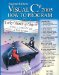# Introduction

Searching data involves determining whether a value (referred to as the search key) is present in the data and, if so, finding the value's location. Two popular search algorithms are the simple linear search and the faster, but more complex, binary search. Sorting places data in order, based on one or more sort keys. A list of names could be sorted alphabetically, bank accounts could be sorted by account number, employee payroll records could be sorted by social security number and so on. This chapter introduces two simple sorting algorithms, the selection sort and the insertion sort, along with the more efficient, but more complex, merge sort. Figure 24.1 summarizes the searching and sorting algorithms discussed in this book.

Figure 24.1. Searching and sorting capabilities in this text.

Chapter

Algorithm

Location

Searching Algorithms:

24

Linear Search

Section 24.2.1

Binary Search

Section 24.2.2

Recursive Linear Search

Exercise 24.8

Recursive Binary Search

Exercise 24.9

27

BinarySearch method of class Array

Fig. 27.3

Contains method of classes ArrayList and Stack

Fig. 27.4

ContainsKey method of class HashTable

Fig. 27.7

Sorting Algorithms:

24

Selection Sort

Section 24.3.1

Insertion Sort

Section 24.3.2

Recursive Merge Sort

Section 24.3.3

Bubble Sort

Exercises 24.524.6

Bucket Sort

Exercise 24.7

Recursive Quicksort

Exercise 24.10

24, 27

Sort method of classes Array and ArrayList

Fig. 24.4,
Figs. 27.327.4

### Searching AlgorithmsVisual C# 2005 How to Program (2nd Edition)
ISBN: 0131525239
EAN: 2147483647
Year: 2004
Pages: 600

Similar book on Amazon